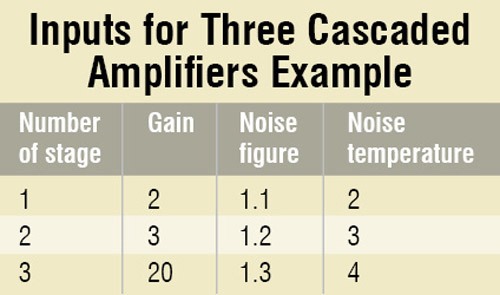## Thursday, 8 March 2018

Parameters like noise, signal-to-noise ratio and gain play an important role in the performance of communication systems. Here we explain these terms and their calculation using MATLAB (matrix laboratory) software.
Gain and noise basics
Gain is a measure of the ability of a circuit (often an amplifier) to increase the power or amplitude of a signal from the input to the output. Noise deteriorates the quality of the received signal (in case of analogue systems) and degrades the throughput (in case of digital systems). Therefore it is important to maximise signal-to-noise ratio (SNR) in a communication system.
The noise figure (NF) is a measure of the degradation of the SNR. In cascaded systems, total noise factor depends upon the gain and NF of each block (or stage). For example, in the case of amplifiers connected in cascade, each amplifier will add its own noise. Hence the overall noise factor will be due to the contribution by each amplifier.
Fig. 1 shows the cascade connection of two amplifiers with noise figures f1 and f2 and gains g1 and g2, respectively.
The noise contribution of each successive stage in a cascaded system is smaller and smaller. The noise of the first stage is the most important. Thus every communication system employs a low-noise amplifier (LNA) at the front to relax the noise requirements. A typically good LNA may have gain of 20 dB and NF less than 1.5 dB.
Noise figure provides a simple method to determine the impact of system noise on sensitivity. Noise temperature is used to express the level of available noise power introduced by a component or source.In this project, MATLAB software is used to design a calculator for the calculation of noise factors. Such type of calculators can be easily created by playing with the array and matrix properties of MATLAB, and can be used for any cascade system with any number of stages. Coding is developed by using simple commands (without using any typical command from MATLAB library).